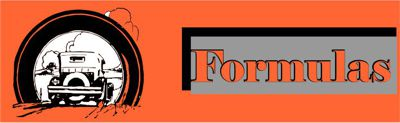A general description of a formula states that variables and symbols are used to show how quantities relate to one another. For any given formula, numbers must be substituted for the variables, to find the value of any specific part of that formula.

 The formula for distance will serve as a good example. Distance equals rate multiplied by time. d = r tA car travels at a rate of 65 mph for 5 hours. How far did it travel? d = 65 x 5 d = 325 milesClara rides her bike for 2.5 hours at a speed of 6.5 mph. How far did Clara travel?